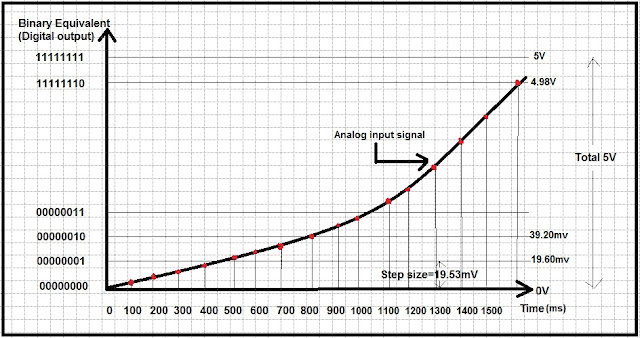# ADC Analog to Digital Converter

2. How to calculate digital value from analog.
3. What is role of reference voltage, resolution, sampling frequency.

## 1. Introduction :

All quantities are physical in real world e.g. temperature, humidity, pressure etc. sensor converts physical quantities to analog quantities like voltage or current. Digital computers or, micro-controllers only understands digital values i.e 0 or 1. Therefore ADCs i.e. analog to digital converters are required, so that micro-controllers can understand digital representation of analog values and we as a programmer can use to build logic.

For an ADC input is analog signal and output is digital value correspond to analog signal. To convert analog signal to digital ADC took help of reference voltage i.e. some known voltage against which ADCs internal circuitry can compare input signal to calculate output digital value. Clock is required for sampling of input data. Star of conversion is either hardware based or software based to star ADC and there will be end of conversion signal flag or interrupt.Fig. 1 ADC Block DiagramFig 2. ADC Conversion

In this topic we will see how do ADC come up with digital value equivalent to Analog signal and role of sampling frequency and resolution of ADC.

#### Role of Sampling Frequency:

When you apply input analog signal to ADC, first thing what ADC do is, it samples input signal. How fast it samples the input signal depends on sampling frequency.  If sampling frequency is 10 HZ then in 1 second ADC will take 10 samples of input signal. Therefore every 100ms (1/10Hz=0.1sec=100ms) ADC will take 1 sample of input signal as shown in Fig 2. More the sampling frequency, more the samples collected which will help to capture variation in input signal precisely. If sampling frequency is less then ADC will not able capture input analog signal properly and variations will be lost. Sampling frequency should be selected as per Nyquist criteria (Sampling frequency must be two times the maximum frequency component of input signal ).

#### Role of Resolution and Reference voltage :

Lets assume ADC is of 8 bit, so what does it means? 8bit ADC means it can output  2^8=256 different digital values 00000000b to 11111111b (0 to 255). Consider reference voltage is 5V. Therefore to represent  256 different output values i.e 0 to 255 , 5V is divided into 255 steps and one step size is  5V/255=19.60mV. Now ADC is able to detect 19.53mV change in input signal. For every change of 19.60V in input signal there is one bit change in digital output. Step size defines the resolution of ADC.  More the resolution lower is the step size, so that ADC will able to detect small change in input signal.  As reference voltage is 5V. Maximum input voltage that can be applied to ADC is 5V.

#### Analog to Digital value calculation :

Consider fig 2. ADC used is 8 bit. Reference voltage is 5V. Sampling frequency 10Hz. Every 100ms ADC will sample input signal. Now this sampled input signal is converted to digital by ADC. Digital value of 100th ms sample is 00000000. When analog input voltage exceeds over 19.60mv and less than 39.2mV, ADC will detect there is change of 19.60mV and represent signal into digital form by 00000001 (sample at 600ms). Similarly digital value of sample taken at 1100ms 00000011.

Dout= Vin/Step Size
Step size= (Vref/((2^n)-1))
where, n is size of ADC e.g For 8 bit ADC n=8.